# OpenGL-ES-案例12：灰度+颠倒+马赛克（3种）滤镜• 准备工作的代码与OpenGL ES 案例11：分屏滤镜中一致，只需要修改相应的底部item数组及对应的着色器名称等，这里不再说明这部分内容
• 顶点着色器也没有任何变化，主要是片元着色器中的实现滤镜算法

## 灰度滤镜

• 权值法：处理后的图片比较逼真
• 浮点算法：`Gray = R*0.3 + G*0.59 + B*0.11` (RGB的权重总和为1)
• 整数方法：`Gray = （R*30 + G*59 + B*11）/100`（RGB的权重总和为100)
• 移位方法：`Gray = （R*76 + G*151 + B*28）>>8`
• 平均值法：`Gray = （R+G+B）/3`，处理后的图片比较柔和
• 仅取绿色：`Gray = G`，这种方式方便简单，且易用

• 浮点算法：这里的RGB权重取自GPUImage框架
``````precision highp float;
uniform sampler2D Texture;
varying vec2 TextureCoordsVarying;

//RGB的变换因子，即权重值
const highp vec3 W = vec3(0.2125, 0.7154, 0.0721);

void main(){
//获取对应纹理坐标系下色颜色值

gl_FragColor = vec4(vec3(luminance), 1.0);
}

• 仅取绿色
``````precision highp float;
uniform sampler2D Texture;
varying vec2 TextureCoordsVarying;

void main(){
//获取对应纹理坐标系下色颜色值

//将RGB全部设置为G，即GRB全部取绿色值
}## 颠倒滤镜

``````precision highp float;
uniform sampler2D Texture;
varying vec2 TextureCoordsVarying;

void main(){

vec4 mask = texture2D(Texture, vec2(TextureCoordsVarying.x, 1.0-TextureCoordsVarying.y));
}

## 马赛克滤镜

• 正方形
• 六边形
• 三角形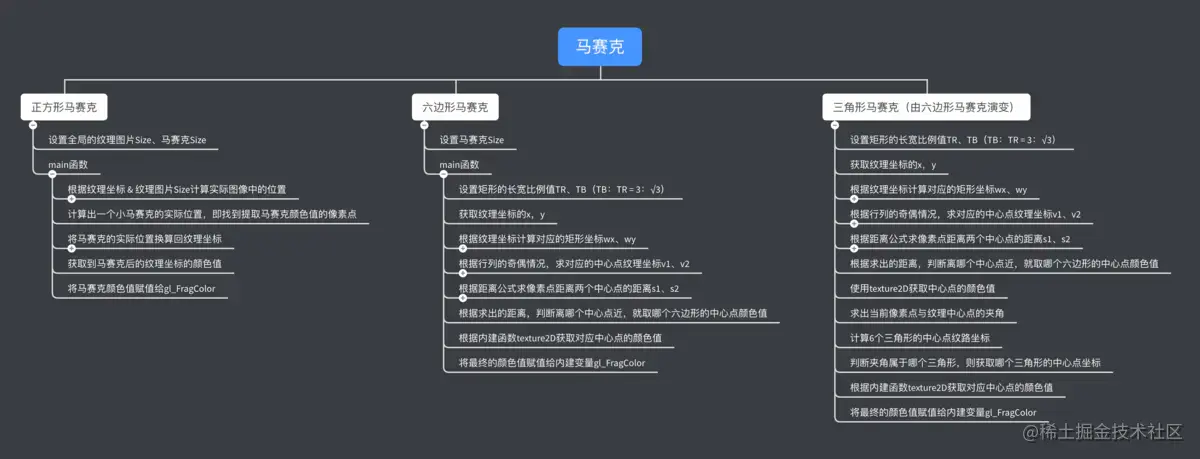### 正方形马赛克

• 根据纹理坐标计算实际图像中的位置，相当于将纹理颜色区放大

• 计算出一个小马赛克的坐标，即找到马赛克提取颜色值的像素点

• 将马赛克坐标换算回纹理坐标，即将纹理颜色区缩小 如图所示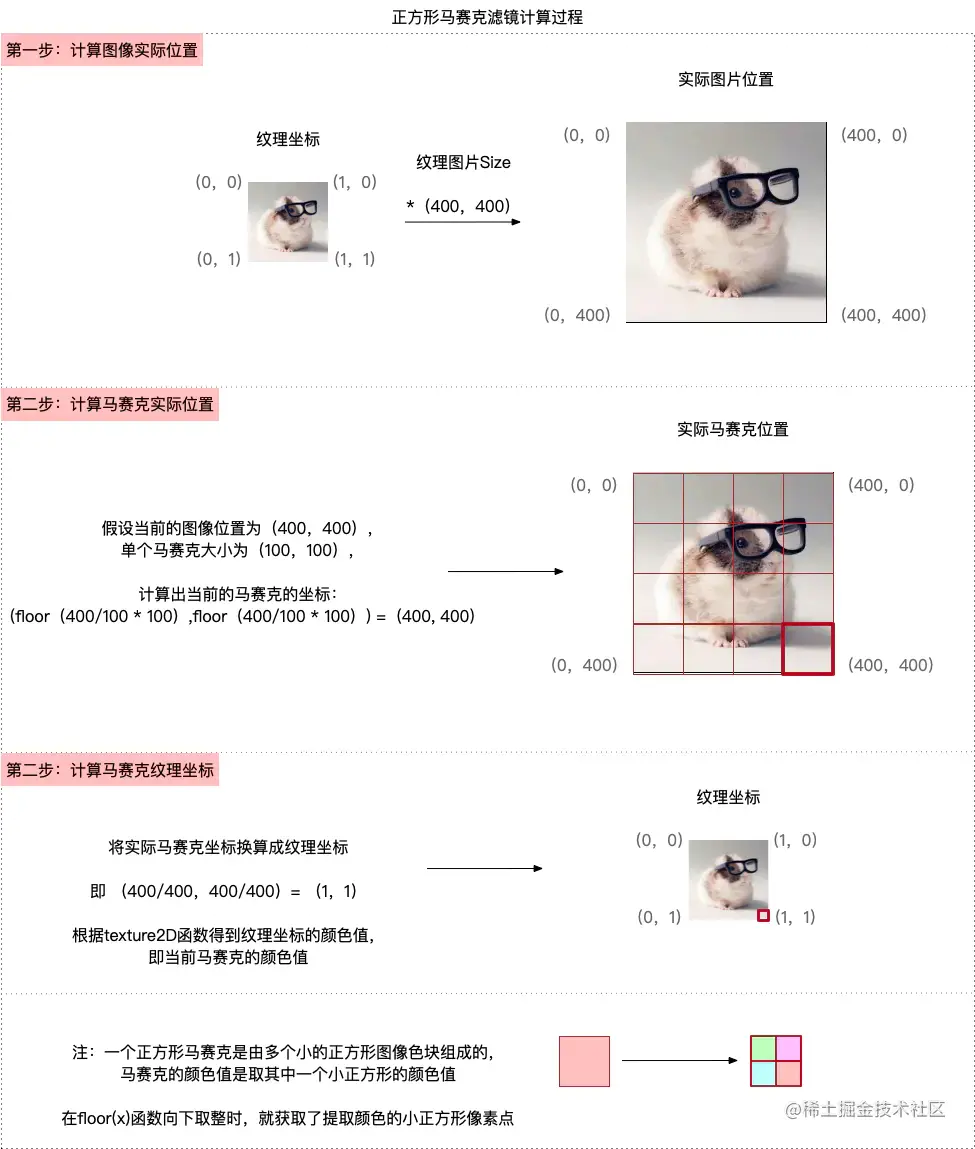``````precision highp float;
//纹理坐标
uniform sampler2D Texture;
//纹理采样器
varying vec2 TextureCoordsVarying;
//纹理图片size
const vec2 TexSize = vec2(400.0, 400.0);
//马赛克size
const vec2 MosaicSize = vec2(16.0, 16.0);

void main(){
//计算实际图像位置
vec2 intXY = vec2(TextureCoordsVarying.x * TexSize.x, TextureCoordsVarying.y * TexSize.y);

//floor(x) 内建函数，返回小于/等于x最大的整数，即向下取整
//floor(intXY.x/mosaicSize.x)*mosaicSize.x 计算出一个小马赛克的坐标
vec2 XYMosaic = vec2(floor(intXY.x/MosaicSize.x)*MosaicSize.x, floor(intXY.y/MosaicSize.y)*MosaicSize.y);

//换算回纹理坐标，此时的纹理坐标是小马赛克的部分的纹理坐标，即某一个色块
vec2 UVMosaic = vec2(XYMosaic.x/TexSize.x, XYMosaic.y/TexSize.y);
//获取到马赛克后的纹理坐标的颜色值
vec4 color = texture2D(Texture, UVMosaic);
//将马赛克颜色值赋值给gl_FragColor
gl_FragColor = color;
}

### 六边形马赛克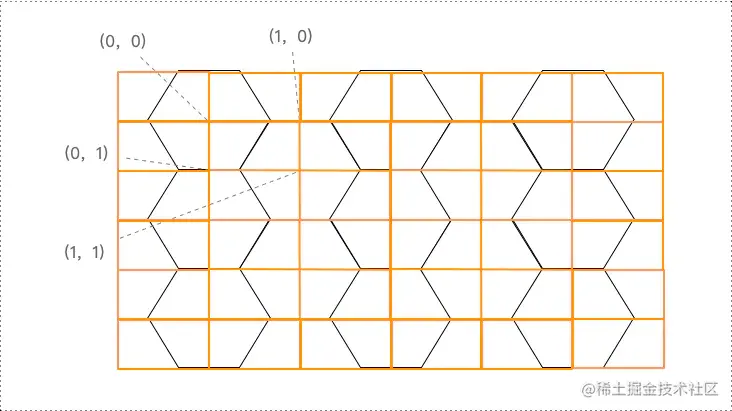• 设置矩形的长宽比例值TR、TB（TB：TR 符合比例 3：√3） 其中长宽比为`3：√3`，计算过程如下：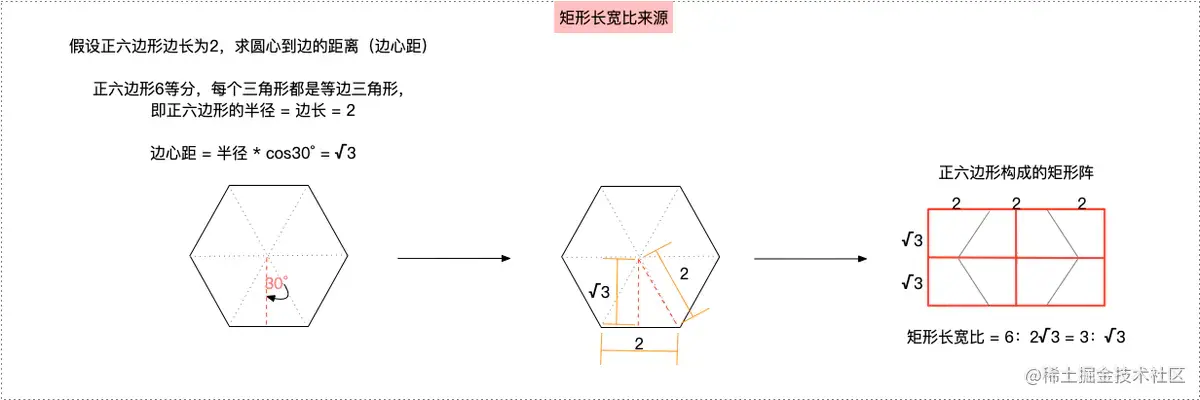• 获取纹理坐标的x，y

• 根据纹理坐标计算对应的矩形坐标wx、wy 假设矩阵的比例为`3*len：√3*len`，那么纹理坐标（x，y）对应的矩阵坐标为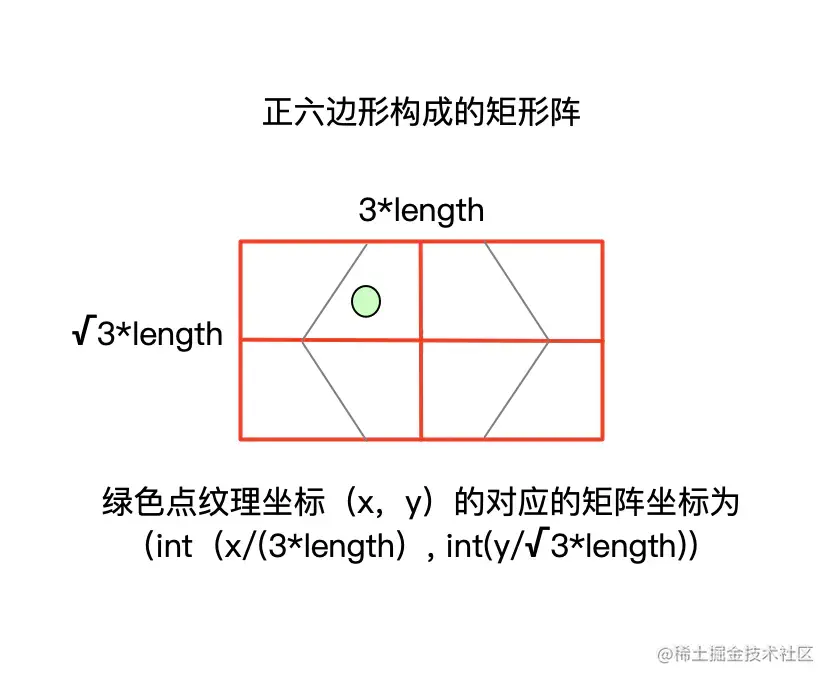• 根据行列的奇偶情况，求对应的中心点纹理坐标v1、v2

• 偶行偶列：（0，0）（1，1）/，即左上、右下

• 偶行奇列：（0，1）（1，0）\，即左下、右上

• 奇行偶列：（0，1）（1，0）\，即左下、右上

• 奇行奇列：（0，0）（1，1）/，即左上、右下 最终汇总起来也只有2种情况，（0，0）（1，1） 和 （0，1）（1，0），如下图所示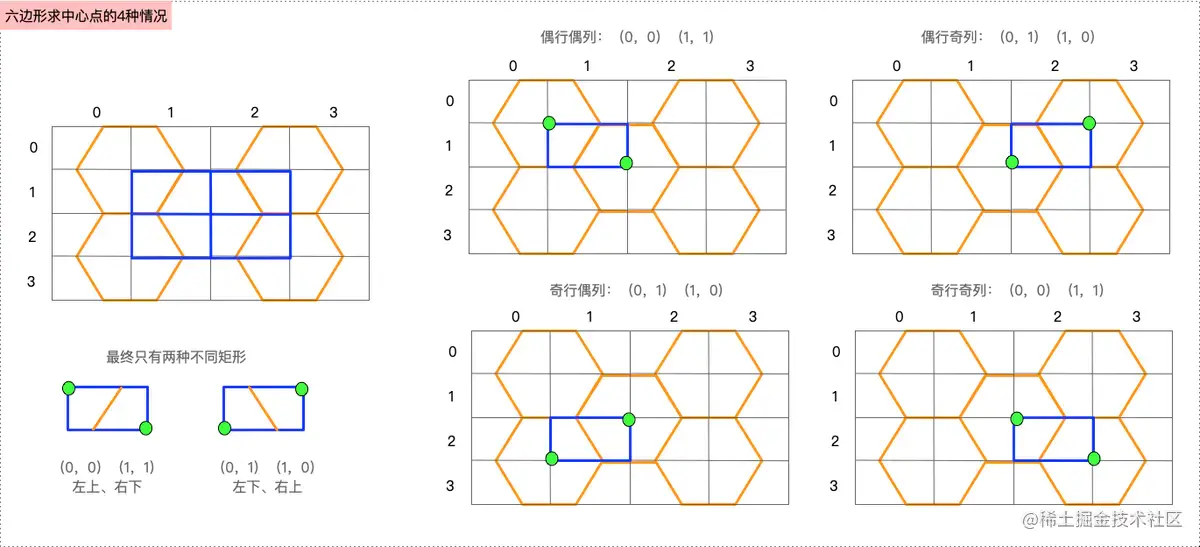• 对于计算中的wx+1，拿（1，0）点来说，wx+1等同于（1，0）与（0，0）之间相差一个矩形的长，这个长度为1，为了得到（1，0）点的坐标，要在（0，0）点坐标的基础上，将wx增加一个长
• 对于计算中的wy+1，拿（0，1）点来说，wy+1等同于（0，0）与（0，1）之间相差一个矩形的高，这个长度为1，为了得到（0，1）点的坐标，要在（0，0）点坐标的基础上，将wy增加一个高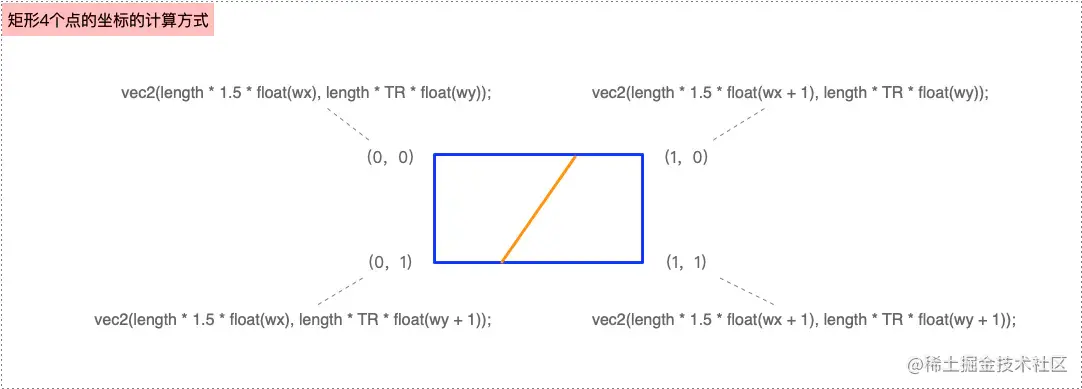• 根据距离公式求像素点距离两个中心点的距离s1、s2

• s1 = √（（v1.x-x）² + （v1.y-y）²）

• s2 = √（（v2.x-x）² + （v2.y-y）²） 如图所示，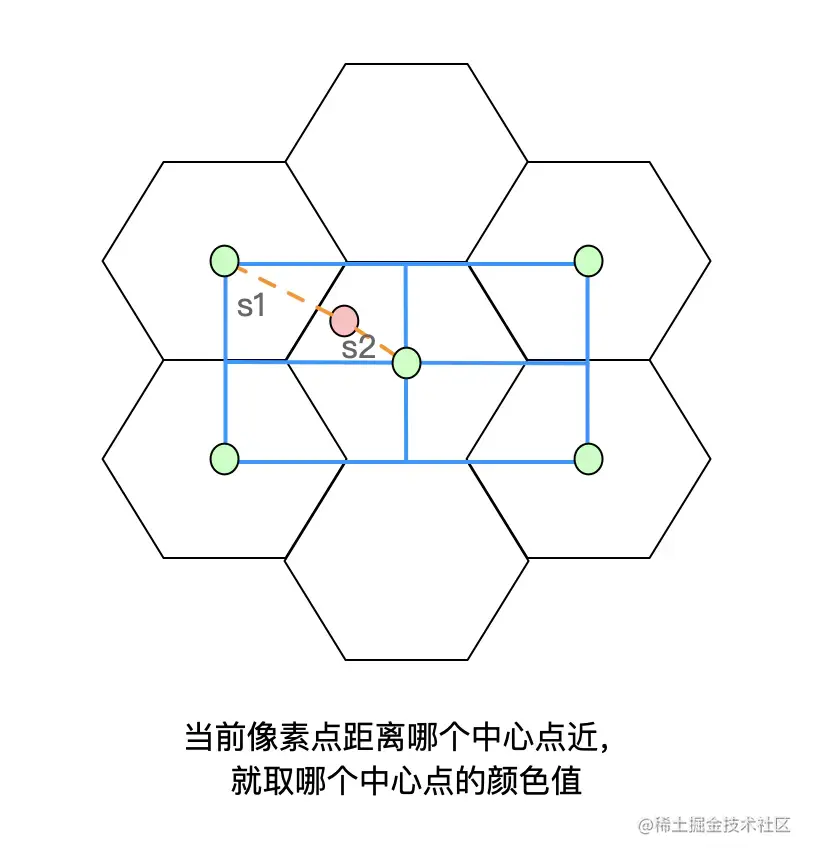• 根据求出的距离，判断离哪个中心点近，就取哪个六边形的中心点颜色值为六边形的颜色值

``````precision highp float;
uniform sampler2D Texture;
varying vec2 TextureCoordsVarying;
//六边形的边长
const float mosaicSize = 0.03;

void main(){

float length = mosaicSize;
//矩形的高的比例为√3，取值 √3/2 ，也可以直接取√3
float TR = 0.866025;
//矩形的长的比例为3，取值 3/2 = 1.5，也可以直接取3
float TB = 1.5;

//取出纹理坐标
float x = TextureCoordsVarying.x;
float y = TextureCoordsVarying.y;

//根据纹理坐标计算出对应的矩阵坐标
//即 矩阵坐标wx = int（纹理坐标x/ 矩阵长），矩阵长 = TB*len
//即 矩阵坐标wy = int（纹理坐标y/ 矩阵宽），矩阵宽 = TR*len
int wx = int(x / TB / length);
int wy = int(y / TR / length);
vec2 v1, v2, vn;

//判断wx是否为偶数，等价于 wx % 2 == 0
if (wx/2 * 2 == wx) {
if (wy/2 * 2 == wy) {//偶行偶列
//(0,0),(1,1)
v1 = vec2(length * TB * float(wx), length * TR * float(wy));
v2 = vec2(length * TB * float(wx+1), length * TR * float(wy+1));
}else{//偶行奇列
//(0,1),(1,0)
v1 = vec2(length * TB * float(wx), length * TR * float(wy+1));
v2 = vec2(length * TB * float(wx+1), length * TR * float(wy));
}
}else{
if (wy/2 * 2 == wy) {//奇行偶列
//(0,1),(1,0)
v1 = vec2(length * TB * float(wx), length * TR * float(wy+1));
v2 = vec2(length * TB * float(wx+1), length * TR * float(wy));
}else{//奇行奇列
//(0,0),(1,1)
v1 = vec2(length * TB * float(wx), length * TR * float(wy));
v2 = vec2(length * TB * float(wx+1), length * TR * float(wy+1));
}
}
//利用距离公式，计算中心点与当前像素点的距离
float s1 = sqrt(pow(v1.x-x, 2.0) + pow(v1.y-y, 2.0));
float s2 = sqrt(pow(v2.x-x, 2.0) + pow(v2.y-y, 2.0));

//选择距离小的则为六边形的中心点，且获取它的颜色
vn = (s1 < s2) ? v1 : v2;
//获取六边形中心点的颜色值
vec4 color = texture2D(Texture, vn);
gl_FragColor = color;
}

### 三角形马赛克

• 求出当前像素点与纹理中心点的夹角 如下图所示，纹理坐标为（x，y），中心点为vn，求夹角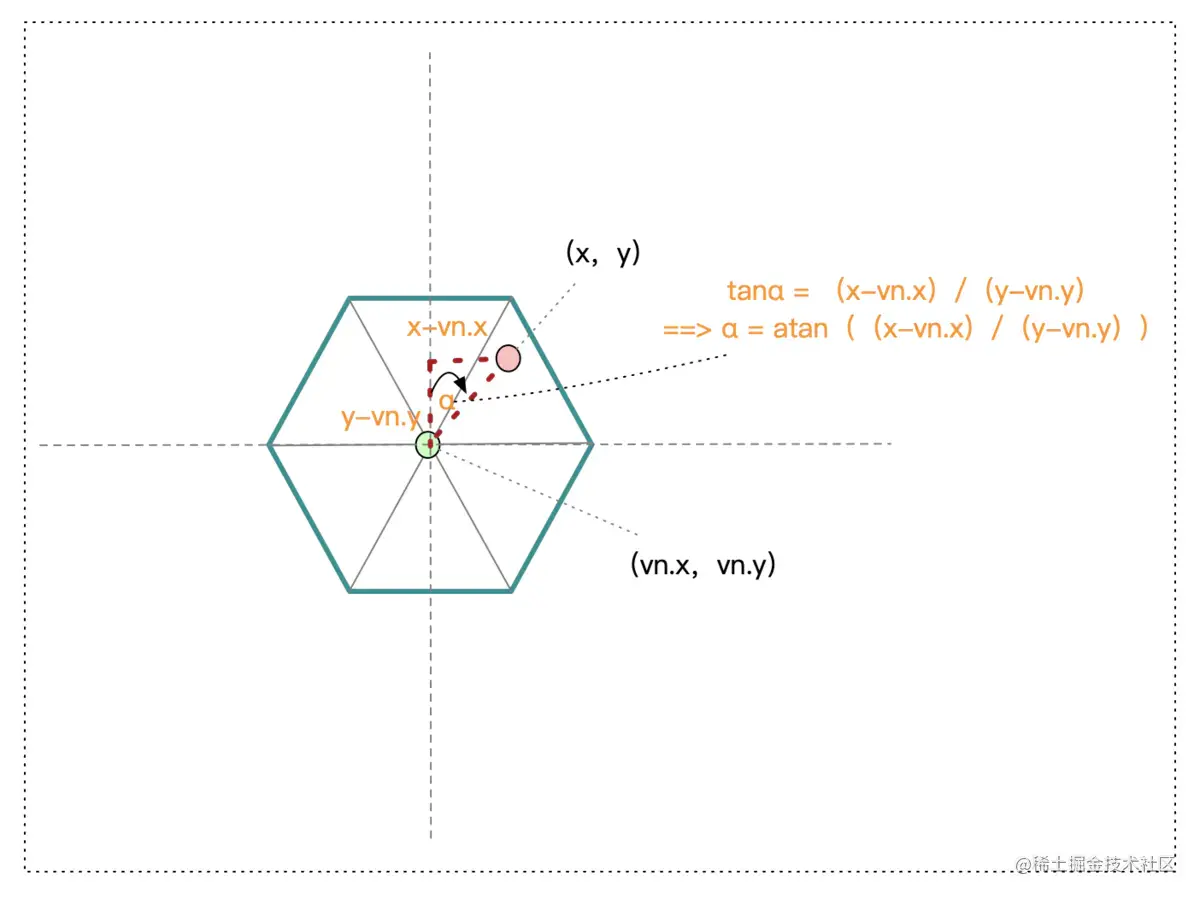• 计算6个三角形的中心点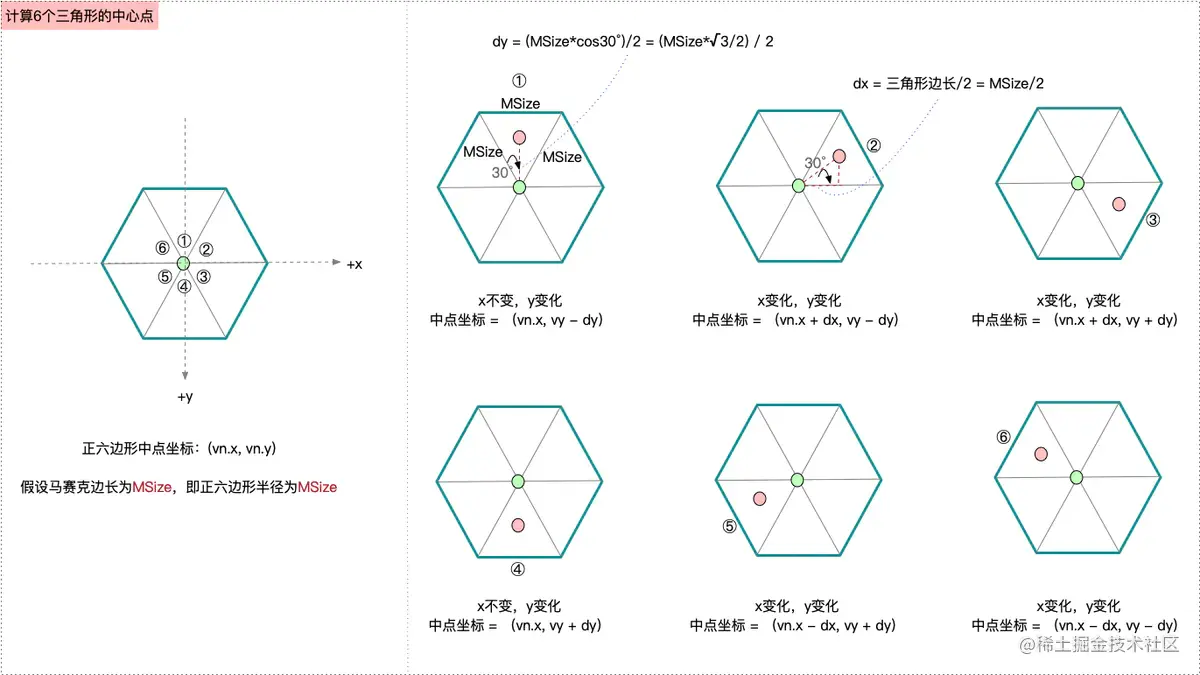• 判断夹角属于哪个三角形，则获取哪个三角形的中心点坐标 其中，不同三角形的夹角范围如图所示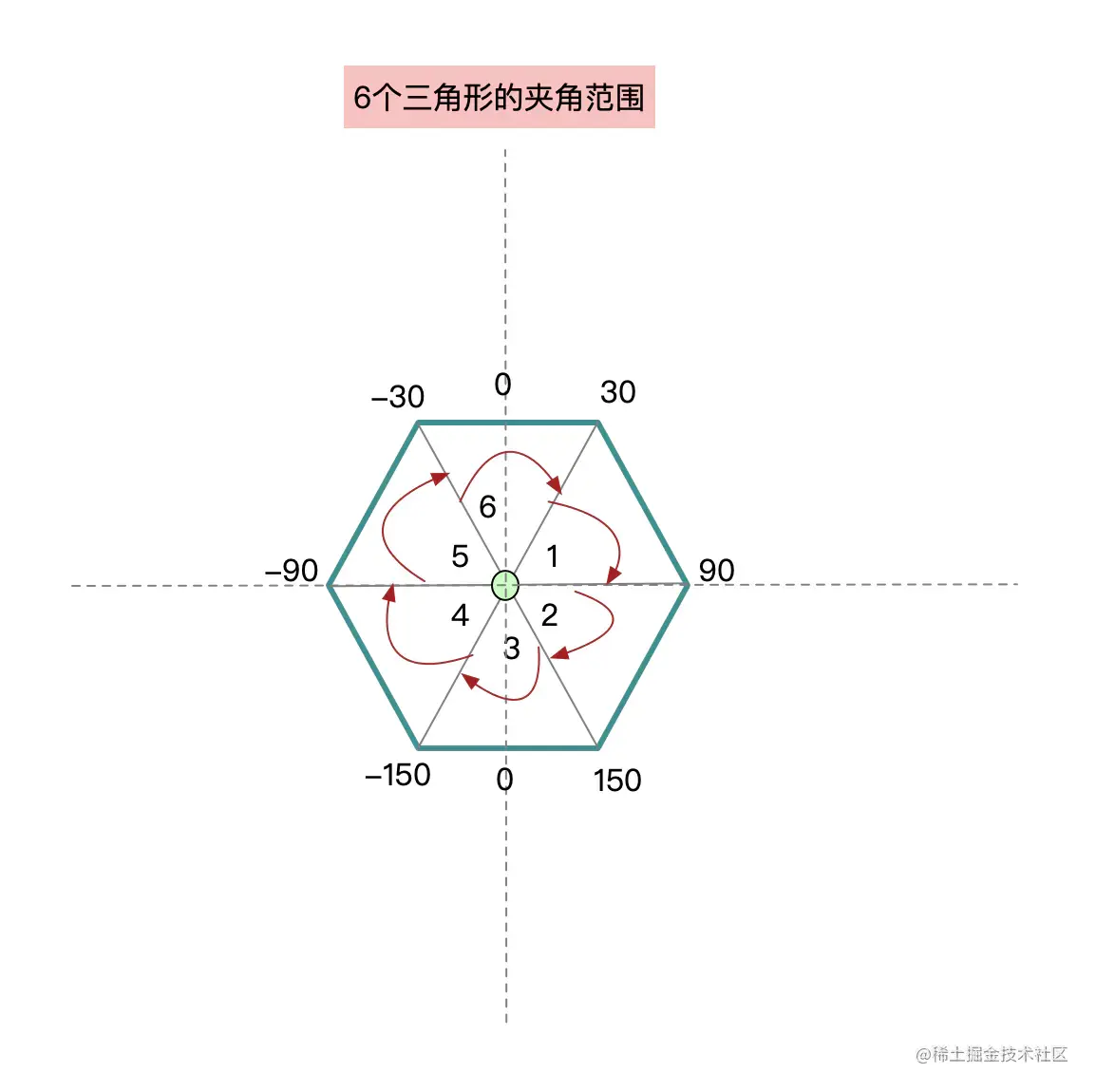``````    //获取像素点与中心点的角度
float a = atan((x-vn.x)/(y-vn.y));

//判断夹角，属于哪个三角形，则获取哪个三角形的中心点坐标
vec2 area1 = vec2(vn.x, vn.y - mosaicSize * TR / 2.0);
vec2 area2 = vec2(vn.x + mosaicSize / 2.0, vn.y - mosaicSize * TR / 2.0);
vec2 area3 = vec2(vn.x + mosaicSize / 2.0, vn.y + mosaicSize * TR / 2.0);
vec2 area4 = vec2(vn.x, vn.y + mosaicSize * TR / 2.0);
vec2 area5 = vec2(vn.x - mosaicSize / 2.0, vn.y + mosaicSize * TR / 2.0);
vec2 area6 = vec2(vn.x - mosaicSize / 2.0, vn.y - mosaicSize * TR / 2.0);

if (a >= PI6 && a < PI6 * 3.0) {
vn = area1;
}else if (a >= PI6 * 3.0 && a < PI6 * 5.0){
vn = area2;
}else if ((a >= PI6 * 5.0 && a <= PI6 * 6.0) || (a < -PI6 * 5.0 && a > -PI6 * 6.0)){
vn = area3;
}else if (a < -PI6 * 3.0 && a >= -PI6 * 5.0){
vn = area4;
}else if (a <= -PI6 && a > -PI6 * 3.0){
vn = area5;
}else if (a > -PI6 && a < PI6){
vn = area6;
}
//获取对应三角形重心的颜色值
vec4 color = texture2D(Texture, vn);
// 将颜色值填充到片元着色器内置变量gl_FragColor
gl_FragColor = color;

atan是GLSL中的内建函数，有两种计算方式 1、atan(y,x) 值域是[0，π], 2、atan(y/x),值域是[-π/2, π/2]